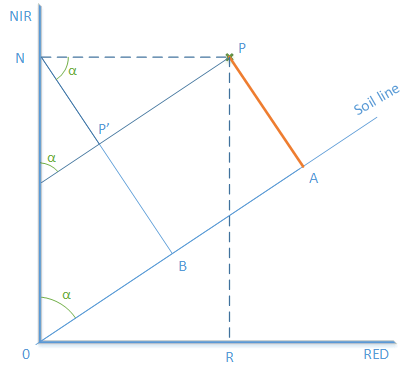# PVI index. Which formula is right?

Hi everyone,

According to SNAP’s help PVI has PVI = sin(a) * IR_factor * near_IR - cos(a) * red_factor * red formula.
According to https://www.asprs.org/wp-content/uploads/pers/1977journal/dec/1977_dec_1541-1552.pdf (the original article where this index was proposed) it has PVI = sqrt((Rgg5-Rp5)2 + (Rgg7-Rp7)^2), formula,
or according to http://www.mdpi.com/2072-4292/4/12/3892/htm#b31-remotesensing-04-03892
it has (pNIR –α pred – b)/(1+α2)
pNIR,soil = α pred,soil+b formula.
There is no sin or cos in initial formula? Why does SNAP use its own formula?

Hello.

This formula is equivalent to the one proposed in the original article. Here’s why:In the above, PA is the PVI.
PA = P’B
P’B = NB - NP’ => PA = NB - NP’
But:
NB = ON * sin(α) = NIR_value * sin(α)
NP’ = NP * cos(α) = OR * cos(α) = RED_value * cos(α)
Hence:
PA = NIR_value * sin(α) - RED_value * cos(α)

In the original article, PVI = sqrt((Rgg5 - Rp5)^2 + (Rgg7 - Rp7)^2)
Rgg5 and Rgg7 are the coordinates of the A point in the figure above.
Rp5 and Rp7 are the coordinates of the P point in the figure above.
Then, the sqrt formula is, in fact, the distance between the points P and A, which is, in our case, the PA segment.
What has been introduced in SNAP are the weights of the two values (NIR, RED). Using the default value of 1.0 leads to the original formula.

Hope this clears things for you.

Cheers.

2 Likes

Thank you very much, Kraftek

Dear Kraftek,
I have a trouble with calculation a (alpha), I have obtained the soil line formula for our case study, it is : NIR= 0.912*RED+0.014
Now, how i know the alpha angle? or how calculate this angle?
I wish, the snap software wanted the slope of soil line and intercept of soil line, like other software.

regards
Ashkan

Hello Ashkan.

You can simply compute the arctangent of delta NIR / delta RED (for example, take the intersection of RED axis and the pair for maximal NIR). Beware that the value of arctangent is in radians, so you need to convert it to degrees.

Regards,
Cosmin.

thank you very much indeed.
I calculated correctly:heart_eyes: